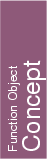## Kernel::ConstructSphere_3

A model for this must provide:

Kernel::Sphere_3
 fo.operator() ( Kernel::Point_3 center, Kernel::FT squared_radius, Orientation orientation = COUNTERCLOCKWISE)
introduces a sphere initialized to the sphere with center center, squared radius squared_radius and orientation orientation.
Precondition: orientationCOPLANAR, and furthermore, squared_radius0.

Kernel::Sphere_3
 fo.operator() ( Kernel::Point_3 p, Kernel::Point_3 q, Kernel::Point_3 r, Kernel::Point_3 s)
introduces a sphere initialized to the unique sphere which passes through the points p, q, r and s. The orientation of the sphere is the orientation of the point quadruple p, q, r, s.
Precondition: p, q, r, and s are not collinear.

Kernel::Sphere_3
 fo.operator() ( Kernel::Point_3 p, Kernel::Point_3 q, Kernel::Point_3 r, Orientation o = COUNTERCLOCKWISE)
introduces a sphere initialized to the smallest sphere which passes through the points p, q, and r. The orientation of the sphere is o.
Precondition: o is not COPLANAR.

Kernel::Sphere_3
 fo.operator() ( Kernel::Point_3 p, Kernel::Point_3 q, Orientation o = COUNTERCLOCKWISE)
introduces a sphere initialized to the smallest sphere which passes through the points p and q. The orientation of the sphere is o.
Precondition: o is not COPLANAR.

Kernel::Sphere_3
 fo.operator() ( Kernel::Point_3 center, Orientation orientation = COUNTERCLOCKWISE)
introduces a sphere s initialized to the sphere with center center, squared radius zero and orientation orientation.
Precondition: orientationCOPLANAR.
Postcondition: s.is_degenerate() = true.

### Refines

AdaptableFunctor (with four arguments)